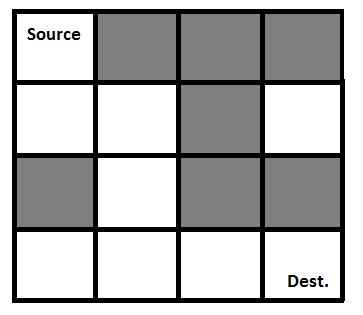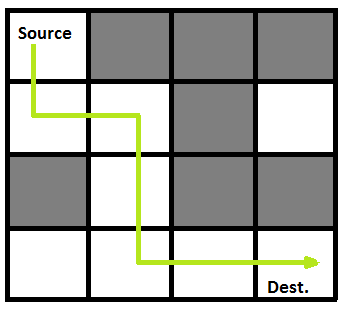Related Articles
Python Program for Rat in a Maze | Backtracking-2
• Last Updated : 11 Dec, 2018

We have discussed Backtracking and Knight’s tour problem in Set 1. Let us discuss Rat in a Maze as another example problem that can be solved using Backtracking.

A Maze is given as N*N binary matrix of blocks where source block is the upper left most block i.e., maze and destination block is lower rightmost block i.e., maze[N-1][N-1]. A rat starts from source and has to reach the destination. The rat can move only in two directions: forward and down.
In the maze matrix, 0 means the block is a dead end and 1 means the block can be used in the path from source to destination. Note that this is a simple version of the typical Maze problem. For example, a more complex version can be that the rat can move in 4 directions and a more complex version can be with a limited number of moves.

Following is an example maze.

` Gray blocks are dead ends (value = 0). `Following is binary matrix representation of the above maze.

```                {1, 0, 0, 0}
{1, 1, 0, 1}
{0, 1, 0, 0}
{1, 1, 1, 1}
```

Following is a maze with highlighted solution path.Following is the solution matrix (output of program) for the above input matrx.

```                {1, 0, 0, 0}
{1, 1, 0, 0}
{0, 1, 0, 0}
{0, 1, 1, 1}
All enteries in solution path are marked as 1.
```

## Python3

 `# Python3 program to solve Rat in a Maze  ` `# problem using backracking  ` ` `  `# Maze size ` `N ``=` `4` ` `  `# A utility function to print solution matrix sol ` `def` `printSolution( sol ): ` `     `  `    ``for` `i ``in` `sol: ` `        ``for` `j ``in` `i: ` `            ``print``(``str``(j) ``+` `" "``, end ``=``"") ` `        ``print``("") ` ` `  `# A utility function to check if x, y is valid ` `# index for N * N Maze ` `def` `isSafe( maze, x, y ): ` `     `  `    ``if` `x >``=` `0` `and` `x < N ``and` `y >``=` `0` `and` `y < N ``and` `maze[x][y] ``=``=` `1``: ` `        ``return` `True` `     `  `    ``return` `False` ` `  `""" This function solves the Maze problem using Backtracking.  ` `    ``It mainly uses solveMazeUtil() to solve the problem. It  ` `    ``returns false if no path is possible, otherwise return  ` `    ``true and prints the path in the form of 1s. Please note ` `    ``that there may be more than one solutions, this function ` `    ``prints one of the feasable solutions. """` `def` `solveMaze( maze ): ` `     `  `    ``# Creating a 4 * 4 2-D list ` `    ``sol ``=` `[ [ ``0` `for` `j ``in` `range``(``4``) ] ``for` `i ``in` `range``(``4``) ] ` `     `  `    ``if` `solveMazeUtil(maze, ``0``, ``0``, sol) ``=``=` `False``: ` `        ``print``(``"Solution doesn't exist"``); ` `        ``return` `False` `     `  `    ``printSolution(sol) ` `    ``return` `True` `     `  `# A recursive utility function to solve Maze problem ` `def` `solveMazeUtil(maze, x, y, sol): ` `     `  `    ``# if (x, y is goal) return True ` `    ``if` `x ``=``=` `N ``-` `1` `and` `y ``=``=` `N ``-` `1``: ` `        ``sol[x][y] ``=` `1` `        ``return` `True` `         `  `    ``# Check if maze[x][y] is valid ` `    ``if` `isSafe(maze, x, y) ``=``=` `True``: ` `        ``# mark x, y as part of solution path ` `        ``sol[x][y] ``=` `1` `         `  `        ``# Move forward in x direction ` `        ``if` `solveMazeUtil(maze, x ``+` `1``, y, sol) ``=``=` `True``: ` `            ``return` `True` `             `  `        ``# If moving in x direction doesn't give solution  ` `        ``# then Move down in y direction ` `        ``if` `solveMazeUtil(maze, x, y ``+` `1``, sol) ``=``=` `True``: ` `            ``return` `True` `         `  `        ``# If none of the above movements work then  ` `        ``# BACKTRACK: unmark x, y as part of solution path ` `        ``sol[x][y] ``=` `0` `        ``return` `False` ` `  `# Driver program to test above function ` `if` `__name__ ``=``=` `"__main__"``: ` `    ``# Initialising the maze ` `    ``maze ``=` `[ [``1``, ``0``, ``0``, ``0``], ` `             ``[``1``, ``1``, ``0``, ``1``], ` `             ``[``0``, ``1``, ``0``, ``0``], ` `             ``[``1``, ``1``, ``1``, ``1``] ] ` `              `  `    ``solveMaze(maze) ` ` `  `# This code is contributed by Shiv Shankar `

Output:

```1 0 0 0
1 1 0 0
0 1 0 0
0 1 1 1
```

Please refer complete article on Rat in a Maze | Backtracking-2 for more details!

My Personal Notes arrow_drop_up
Recommended Articles
Page :